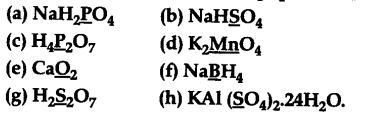# Assign oxidation number to the underlined elements in each of the following species

Assign oxidation number to the underlined elements in each of the following species(a) Let the oxidation no. of P in Na\${{H}{2}}\$P\${{O}{4}}\$ be x.

• 1 + 2(+ 1) + x + 4(- 2) =0
• 1 + 2 + x-8 =0
x = + 5
Oxidation number of P in Na\${{H}{2}}\$P\${{O}{4}}\$ = + 5.
(b) Let the oxidation no. of S in NaHS\${{O}_{4}}\$ be x.
• 1 + 1 + x + 4(- 2) =0
• 2 + x - 8=0
x = + 6
Oxidation number of S in NaHS\${{O}{4}}\$ = + 6.
© Let the oxidation number of P in \${{H}
{4}}\$\${{P}{2}}\$\${{O}{7}}\$ be x.
4(+ 1) + 2x + 7(-2) =0
• 4 + 2x -14 =0
2x =10
x = + 5
Oxidation number of P in \${{H}{4}}\$\${{P}{2}}\$\${{O}{7}}\$ = + 5.
(d) Let the oxidation no. of Mn in \${{K}
{2}}\$Mn\${{O}_{4}}\$ be x.
2(+ 1) + x + 4(- 2) =0
• 2 + x-8 =0
x = + 6
Oxidation number of Mn in \${{K}{2}}\$Mn\${{O}{4}}\$ = + 6.
(e) Let the oxidation number of oxygen in Ca\${{O}_{2}}\$ be x.
• 2 + 2x =0
[oxidation no. of Ca = + 2]
2x = -2
x =-1
Oxidation number of O in Ca\${{O}{2}}\$ =-1.
(f) Let the oxidation no. of B in NaB\${{H}
{4}}\$ be x.
• l+x + 4(-l) =0
[•.• oxidation no. of H = -1]
x-3 =0
x = + 3
Oxidation number of B in NaB\${{H}{4}}\$ = + 3.
(g) Let the Oxidation no. of S in \${{H}
{2}}\$\${{S}{2}}\$\${{O}{7}}\$ be x.
2(+1)+ 2x + 7(-2) =0
• 2 + 2x -14 =0
2x =12
x = + 6
Oxidation number of S in \${{H}{2}}\$\${{S}{2}}\$\${{O}{7}}\$ = + 6.
(h) Let the oxidation no. of S in KAl(S\${{({{O}
{4}})}{2}}\$).24\${{H}{2}}\$O = x
• 1 + 3 + 2x + 8(- 2) + 24(+ 2) + 24(- 2) = 0
• 4 + 2x -16 = 0
[O. No. of K = 1,O. No. of Al = 3]
2x =12
x = +6
Oxidation number of S in KAl(S\${{({{O}{4}})}{2}}\$).24\${{H}_{2}}\$O=+6